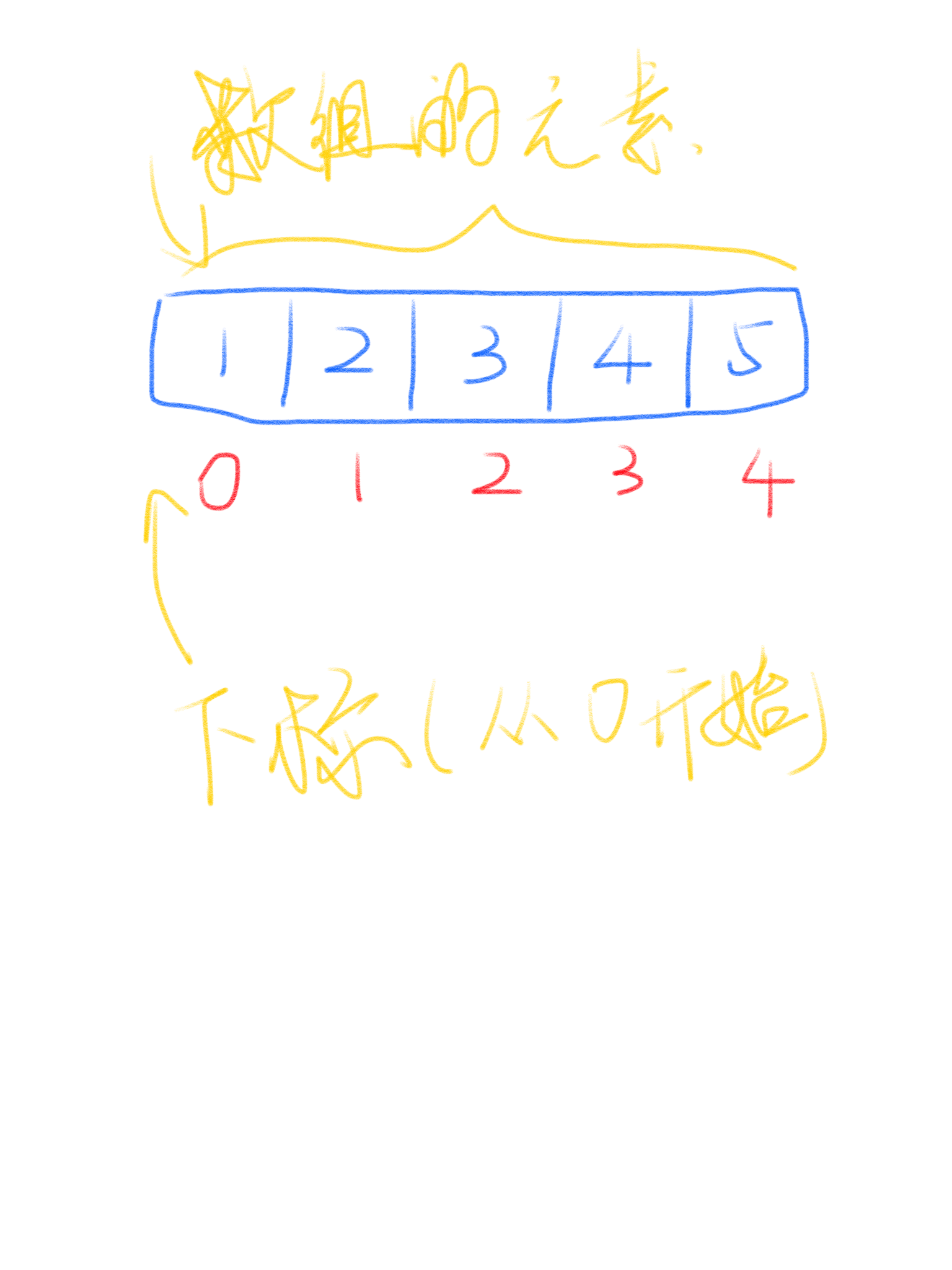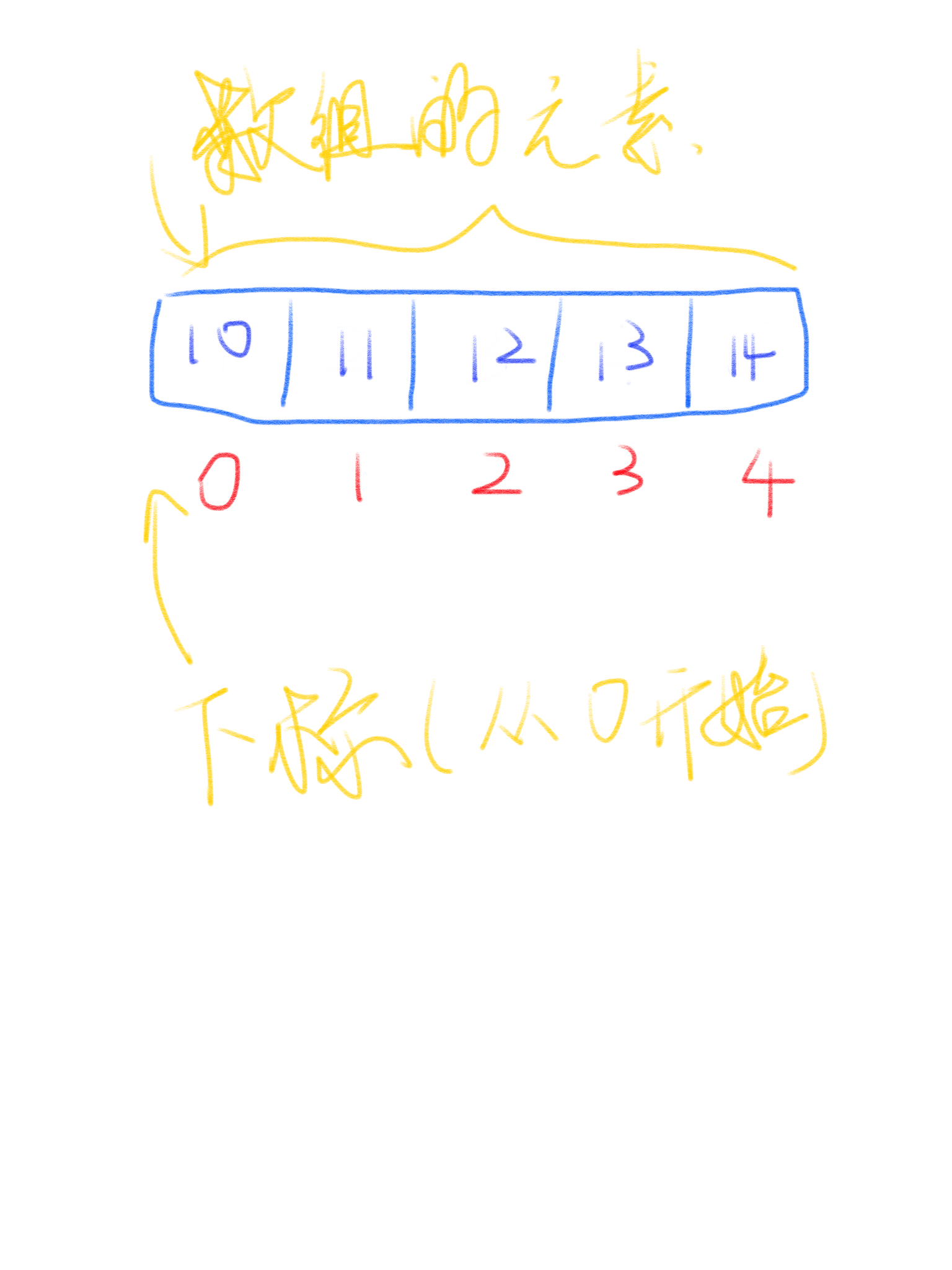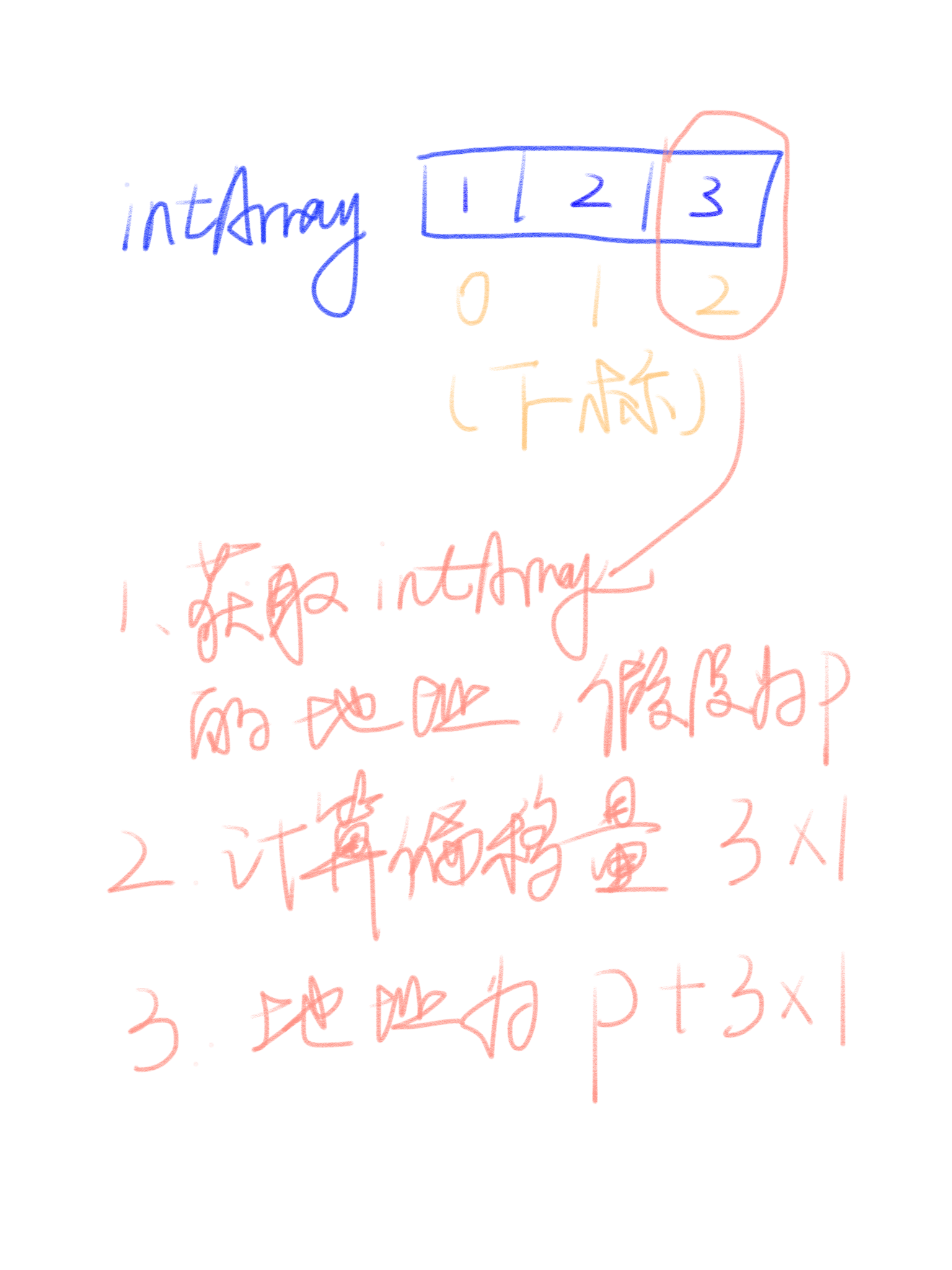# 数组(array)## 数组的使用

• 固定长度
• 支持根据下标来获取元素
``````package main

import (
"fmt"
)

func main() {
var intArray = int{1, 2, 3}

fmt.Printf("%d", intArray)
}
``````## 数组在实际项目中的使用

``````type slice struct {
array unsafe.Pointer
len   int
cap   int
}
``````

``````func Search(n int, f func(int) bool) int {
// Define f(-1) == false and f(n) == true.
// Invariant: f(i-1) == false, f(j) == true.
i, j := 0, n

for i < j {
h := int(uint(i+j) >> 1) // avoid overflow when computing h
// i ≤ h < j
if !f(h) {
i = h + 1 // preserves f(i-1) == false
} else {
j = h // preserves f(j) == true
}
}

// i == j, f(i-1) == false, and f(j) (= f(i)) == true  =>  answer is i.
return i
}
``````

## 总结

• Go语言中slice的实现
• 二分查找中使用数组来存储数据

##### 更多文章
• 欣赏一下K&R两位大神的代码
• MySQL的ON DUPLICATE KEY UPDATE语句
• 使用microk8s快速搭建k8s
• Python中优雅的处理文件路径
• Go语言MySQL时区问题
• 我的技术栈选型
• 为什么我要用Linux作为桌面？
• disqus获取评论时忽略query string
• MySQL性能优化指南
• 网络编程所需要熟悉的那些函数
• DNSCrypt简明教程
• SQLAlchemy简明教程
• 这些年，我们错过的n个亿
• 给Linux用户的FreeBSD快速指南
• 旧电脑也不能闲着：家用备份方案

•##### 微信公众号本站热门